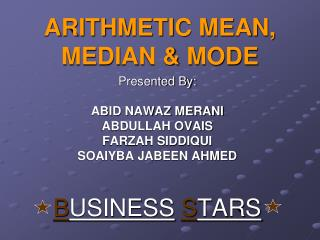# ARITHMETIC MEAN, MEDIAN & MODE - PowerPoint PPT PresentationDownload PresentationARITHMETIC MEAN, MEDIAN & MODE

ARITHMETIC MEAN, MEDIAN & MODE
Download Presentation## ARITHMETIC MEAN, MEDIAN & MODE

- - - - - - - - - - - - - - - - - - - - - - - - - - - E N D - - - - - - - - - - - - - - - - - - - - - - - - - - -
##### Presentation Transcript

1. ARITHMETIC MEAN, MEDIAN & MODE Presented By: ABID NAWAZ MERANI ABDULLAH OVAIS FARZAH SIDDIQUI SOAIYBA JABEEN AHMED BUSINESSSTARS

2. INTRODUCTION TO ARITHMETIC MEAN Given By: Abid Nawaz Merani

3. The raw data given below show the scores of an Australian Batsman of his last 30 matches. 60 71 52 67 89 79 73 49 62 66 78 90 72 58 65 76 81 69 80 57 54 73 61 73 45 51 63 60 76 85 Calculate the Mean, Median & Mode from the above raw data and also draw graph.

4. Calculating RANGE:- Maximum Value – Minimum Value = Range 90 – 45 = 45 Calculating NUMBER OF CLASSES:- No. of Classes = 1 + 3.3 log10(n) = 1 + 3.3 log10(30) = 5.874 or 6 (approx)

5. Calculating WIDTH (h) :- Width = Range No. of Classes Width = 45 5.874 Width = 7.66 or 8 (approx)

6. C - I Tele-Mark f 45 – 52 IIII 4 53 – 60 IIII 5 61 – 68 IIII I 6 69 – 76 IIII III 8 77 – 84 IIII 4 85 – 92 III 3

7. ARITHMETIC MEAN Further Explained By: Farzah Siddqui

8. For Arithmetic Mean:- Sum of the products of frequencies and mid-points, divided by the sum of all frequencies. The Formula of Mean for group data is : X = ∑ f x ∑ f

9. C - I f x fx 45 – 52 4 48.5 194.0 53 – 60 5 56.5 282.5 61 – 68 6 64.5 387.0 69 – 76 8 72.5 580.0 77 – 84 4 80.5 322.0 85 – 92 3 88.5 265.5 Total ∑ f = 30∑ f x = 2031

10. Calculating MID-POINT (x):- Formula for the Mid-point x1 + y1 2 Where, “x1” can be 45, 53, 61, 69,77 or 85 and “y1” can be 52, 60, 68, 76, 84 or 92 respectively. 45 + 52 => 48.5 2

11. Calculating Arithmetic Mean:- X = ∑ f x ∑ f X = 2031 30 X = 67.7

12. MEDIAN Explained By: Soaiyba Jabeen Ahmed

13. FOR MEDIAN:- C - I f C – B C - F 45 – 52 4 44.5 – 52.5 04 53 – 60 5 52.5 – 60.5 09 61 – 68 6 60.5 – 68.5 15 69 – 76 8 68.5 – 76.5 23 77 – 84 4 76.5 – 84.5 27 85 – 92 3 84.5 – 92.5 30 ∑f = 30

14. FORMULA FOR MEDIAN:- In case of frequency distribution: Median = l + h ∑ f – C.F f 2 Median Class:- Median Class = ∑ f = 30 => 15 2 2

15. Where, L = Lower class boundary of Median Class h = Class Height f = Frequency ∑f = Sum of frequency C.F = Cumulative frequency of Preceding class

16. By Putting The Values In The Formula, X = 60.5 + 8 30 – 9 6 2 X = 68.5

17. MODE Explained By: Abdullah Ovais

18. FOR MODE:- C - I f C – B 45 – 52 4 44.5 – 52.5 53 – 60 5 52.5 – 60.5 61 – 68 6 60.5 – 68.5 69 – 76 8 68.5 – 76.5 77 – 84 4 76.5 – 84.5 85 – 92 3 84.5 – 92.5 ∑ f = 30

19. FORMULA FOR MODE:- In case of frequency distribution: Mode = l + fm – f1 h 2fm – f1 – f2

20. Where, L = Lower class boundary of Modal Class h = Class Height Fm = Frequency of modal class / Highest frequency f1 = Preceding frequency of Modal Class f2 = Following frequency of Modal Class

21. By Putting The Values In The Formula, X = 68.5 + 8 – 6 8 2(8) – 6 – 4 X = 71.16 Hence, Mode > Median > Mean 71.16 > 68.5 > 67.7

22. GRAPHICAL REPRESENTAION:- 8-- HISTOGRAM 7-- F R 6-- E Q 5-- U E4-- N C3-- Y 2-- 1-- 44.5 52.5 60.5 68.5 76.5 84.5 92.5 CLASS BOUNDARIES

23. NEGATIVELY SKEWED CURVE:-

24. EXAMPLE OF POSITIVELY SKEWED CURVE Given By: Soaiyba Jabeen Ahmed

25. EXAMPLE OF SYMETRICAL CURVE Given By: Abid Nawaz Merani

26. PRESENTATION ENDED We are very thankful to our respected teacher Mr. Zafar Ali and the students of our class for their precious time and kind attention and for maintaining the discipline throughout the Presentation. Please excuse, if anyone of us hurt you, we really didn’t mean it. READERS ARE THE LEADERS